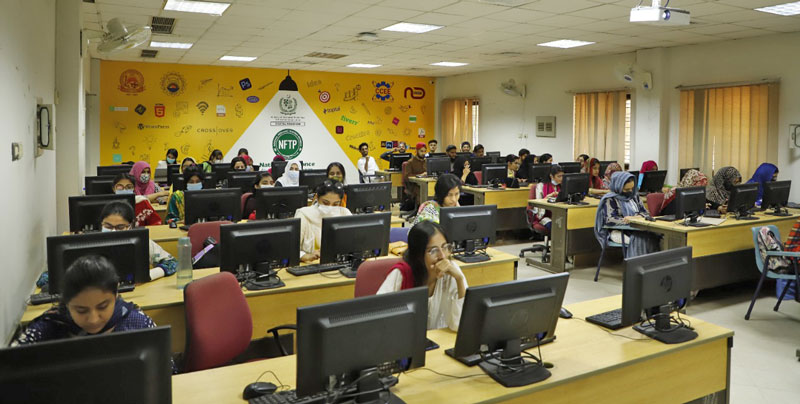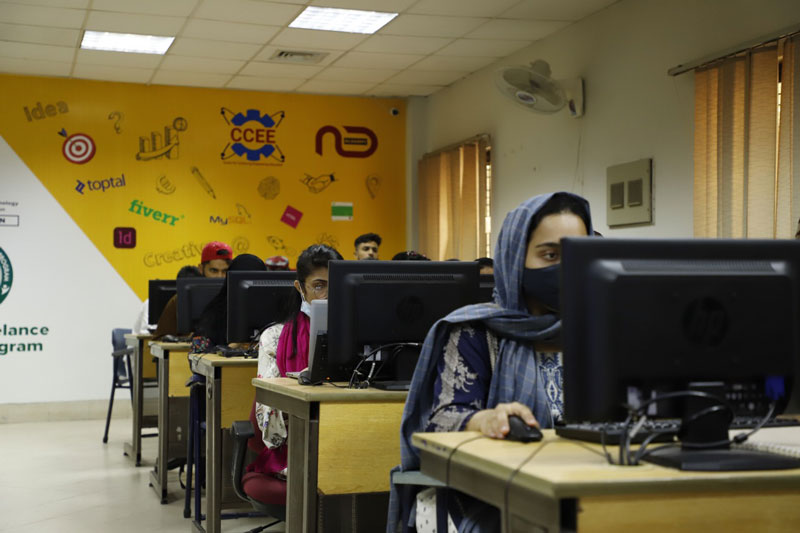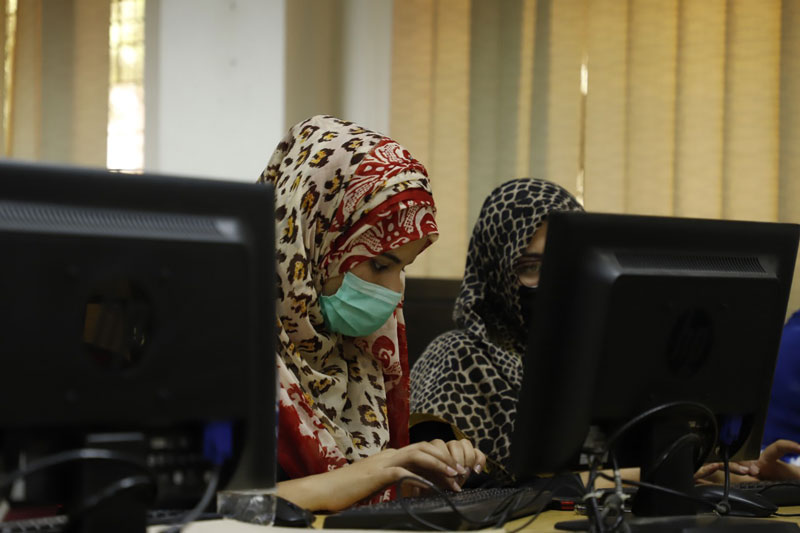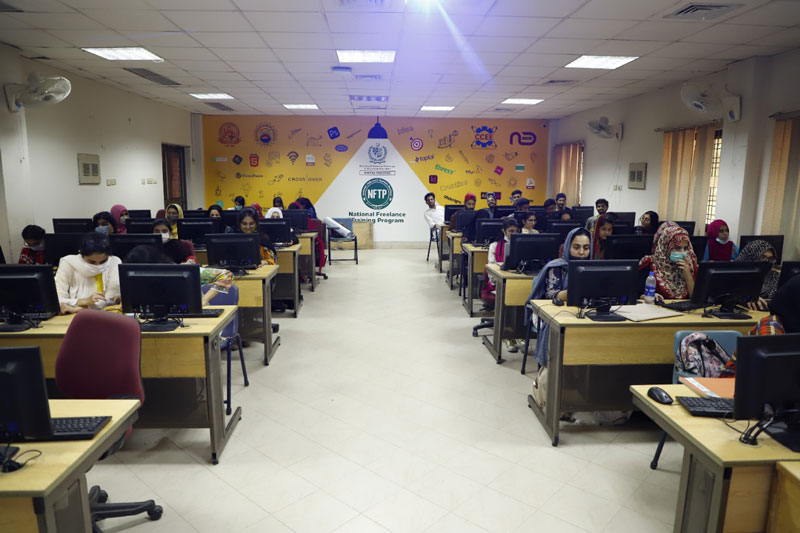#### Success Story of 1st Batch of National Freelance Training Program

Statistics of NFTP 1st Batch DCS Students:

 Total Graduates= 134 (Female=53, Male=81) Content Marketing and advertising=45 (Female=21, Male=24) Technical= 44 (Female=6, Male=38) Creative Design=45 (Female=26, Male=19) Total Earnings= 6100 (Female=699, Male=5401)  Content Marketing and advertising=2470 (Female=73, Male=2397) Technical= 2133 (Female= 200, Male=1933) Creative Design=1497 (Female=426, Male=1071) Total Earners=25 (Female=11, Male=14) Content Marketing and advertising=9 ( Female= 4, Male=5) Technical= 7 (Female= 1, Male=6) Creative Design=9 (Female=6, Male=3)